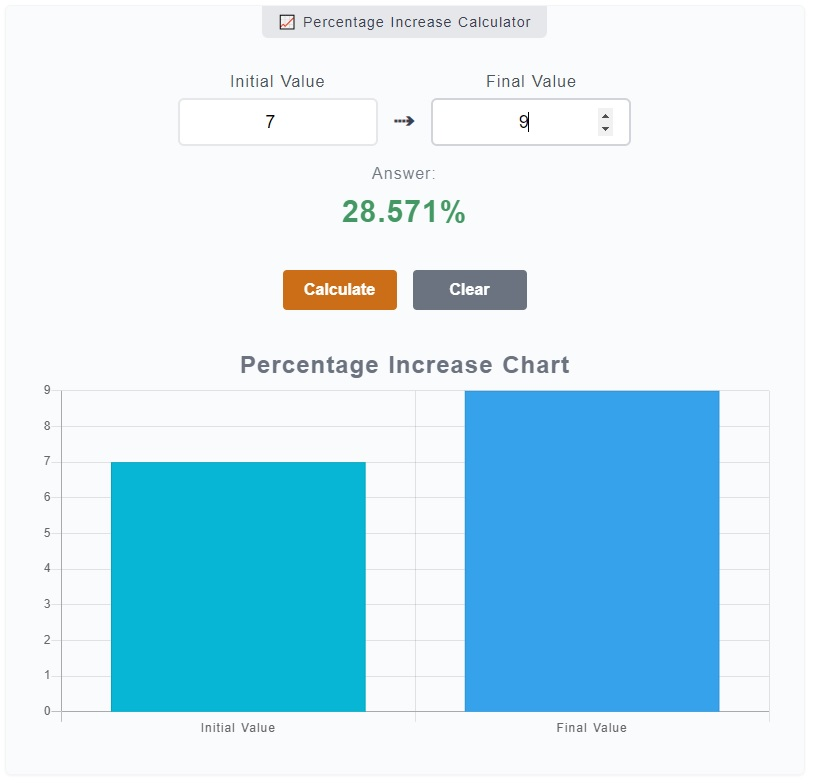# Percentage Increase Calculator

The Percentage Increase Calculator is used for one purpose only, to get the percentage increase of a number from an initial value to the final value. For instance, you want to know what is the percentage increase from intial value of 7 to a final value of 9, this calculator will do the computation for you.

📈 Percentage Increase Calculator

0%

## How to Use the Percentage Increase Calculator

Since the calculator only solves a singular problem, navigating to it's usage would just be a simple task. All you ever need to do is enter the numbers and click on the `Calculate` button. Here's a step by step guide to it:1. Step 1

On the calculator, there are two (2) input fields - initial value and final value. On the initial value, enter the number from it's starting value. Enter the final value on the corresponding field as well. So for example, you want to know the percentage increase from 27 to 33. Enter `27` on `Initial Value` input field and `33` to the `Final Value` input field.

2. Step 2

After you have entered the required inputs, you can just press `Enter` on your keyboard or `Calculate` button.